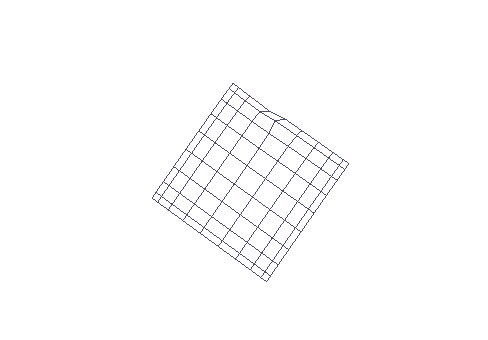A high performance, open source, parallel MMF library written in C++.
Authors:  Risi Kondor, Nedelina Teneva and Pramod K. Mudrakarta.
PRE-RELEASE VERSION
http://people.cs.uchicago.edu/~risi/MMF/index.html

Features:
High performance routines for computing the MMF of any symmetric matrix.
Parallel implementation designed for multicore/multiprocessor architectures.
Fully object oriented design with a transparent API.
MATLAB interface (coming soon).

Requires:
C++11 compatible compiler

Optional:
Eigen or LAPACK for some linear algebra tasks
Matio for Matlab format input/output
OpenGL and GLUT for graphics

View on Github:  [github]
Documentation:  [draft]
Performance:  [gallery]
BibTeX entry:  [bib]
Help/support email:  pMMF.library at gmail.com

### The MMF FAQ

• What is Multiresolution Matrix Factorization (MMF)?

MMF is an algorithm for factorizing any (potentially large) symmetric matrix $$A\in \mathbb{R}^{n\times n}$$ in the form $Q_L \ldots Q_2\, Q_1\hspace{.1em} A\hspace{.1em} Q_1^\top Q_2^\top \ldots Q_L^\top\approx H$ where
• $$Q_1,... Q_L$$ is a sequence of carefully chosen sparse orthogonal matrices (rotations). In the simplest case, each $$Q_\ell$$ is just a Givens rotation.
• The matrix $$H$$ is core-diagonal, meaning that it is zero everywhere except (a) a certain small $$m\times m$$ dimensional block, called its core, and (b) its diagonal.
• The $$Q_1,... Q_L$$ rotations satsify an additional multiresolution constraint with the effect that $A\mapsto Q_1\hspace{.1em} A\hspace{.1em} Q_1^\top\mapsto Q_2\, Q_1\hspace{.1em} A\hspace{.1em} Q_1^\top Q_2^\top \mapsto \ldots$ progressively compresses $$A$$ to smaller and smaller sizes, or, alternatively, provides a sketch of $$A$$ at lower and lower levels of resolution.

• What is MMF used for?

• Matrix compression/sketching by providing a good quality low dimensional approximation to huge matrices, especially those arising in machine learning problems.
• Preconditioning to accelerate the solution of large linear systems.
• Sparse approximation by providing a multiresolution (wavelet) basis for $$A$$.
• Data analysis by helping to uncover the structure of $$A$$ itself, for example, when $$A$$ is the adjacency matrix of a complex network.

• What is Parallel MMF (pMMF)?

pMMF is a fast concurrent algorithm for finding MMF factorizations. Empirially, on many classes of naturally occurring sparse matrices, pMMF runs in time close to linear in $$n$$ (see [gallery]).

• What does the animation at the top of this page show?

The animation shows step-by-step what happens when pMMF is applied to the adjacency matrix of 10x10 square grid. After each rotation, one row/column of $$A$$ is designated a "wavelet" and eliminated from the active part of the matrix, effectively reducing the number of vertices in the graph by one. The graph is embedded in the plane using its two lowest positive eigenvalue eigenvectors.